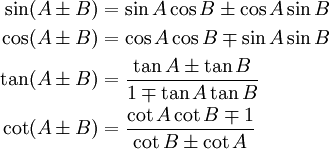# 三角法恒等式

## 直角三角形• θ
• 斜边
• 邻边
• 对边

## 正弦、余弦和正切正弦函数： sin(θ) = 对边 / 斜边 余弦函数： cos(θ) = 邻边 / 斜边 正切函数： tan(θ) = 对边 / 邻边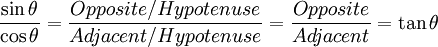tan(θ) = sin(θ)/cos(θ)

## 余割、正割和余切余割函数： csc(θ) = 斜边 / 对边 正割函数： sec(θ) = 斜边 / 邻边 余切函数： cot(θ) = 邻边 / 对边

### 例子：若 对边 = 2，斜边 = 4，则

sin(θ) = 2/4csc(θ) = 4/2

sin(θ) = 1/csc(θ)

cos(θ) = 1/sec(θ)

tan(θ) = 1/cot(θ)

csc(θ) = 1/sin(θ)

sec(θ) = 1/cos(θ)

cot(θ) = 1/tan(θ)

cot(θ) = cos(θ)/sin(θ)

## 勾股定理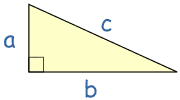勾股定理说：在直角三角形里，a的平方加b的平方等于c的平方： a2 + b2 = c2

a2 c2 = b2 c2 = c2 c2

( a c )2 + ( b c )2 = 1

a/c对边 / 斜边，就是 sin(θ)

b/c邻边 / 斜边，就是 cos(θ)

sin2 θ + cos2 θ = 1

• sin2 θ 的意思是 θ 的正弦的平方，
• sin θ2 的意思是 θ 的平方的正弦

### 例子：32°

• sin(32°) = 0.5299……
• cos(32°) = 0.8480……

sin2 θ + cos2 θ:

0.52992 + 0.84802
= 0.2808…… + 0.7191……
= 0.9999……

sin2 θ = 1 − cos2 θ
cos2 θ = 1 − sin2 θ
tan2 θ + 1 = sec2 θ
tan2 θ = sec2 θ − 1
cot2 θ + 1 = csc2 θ
cot2 θ = csc2 θ − 1

## 怎样去记住这么多的恒等式？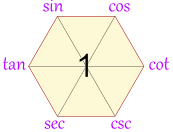## 慢着……还有更多！

### 对角恒等式

sin(−θ) = −sin(θ)

cos(−θ) = cos(θ)

tan(−θ) = −tan(θ)

### 双角恒等式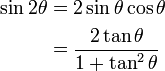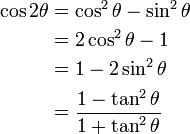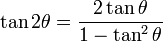### 半角恒等式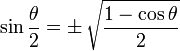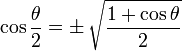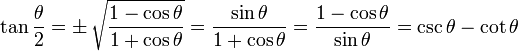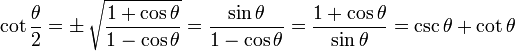### 角度和与角度差恒等式